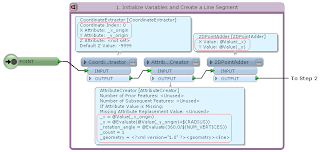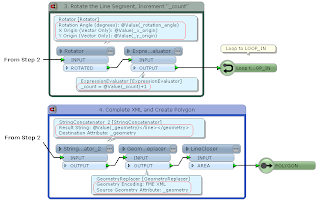## 2013-11-24

### Advanced Geometric Operation: Create Regular Polygons

=====
2013-11-25: Found a custom transformer example called RegularPolygonCreator, the approach is different from my example. FMEpedia > RegularPolygonCreator
=====
This custom transformer example transforms an input point into a regular polygon; number of vertices and radius of the resultant polygon are given through parameters.

The strategy is:
1) Create a line segment directing to north (positive direction of Y axis) from the input point; its length is equal to the given radius.
2) Rotate the line segment by specific angle (= 360 / number of vertices) repeatedly; get coordinate of its end node at each time, transform coordinate values into XML element (<coord x="x value" y="y value" />), and append it to XML fragment.
3) After appending all vertex elements, complete the XML, replace it with a line geometry, and transform the line into a polygon.

For example, the final XML for a triangle should be like this.
-----
<?xml version="1.0" ?>
<geometry>
<line>
<coord x="0" y="0.4" />
<coord x="-0.346410161513776" y="-0.2" />
<coord x="0.346410161513775" y="-0.2" />
</line>
</geometry>
-----
This is in the "FME XML" encoding format, so it can be replaced with a line geometry using the GeometryReplacer transformer. Finally close the line to create a polygon using the LineCloser.Originally, I needed to create radiational lines in an actual project. The example above is derived from that.

=====
2015-10-16: A simpler way to create a regular N-gon.
(1) Create a circle area with the 2DEllipseReplacer.
(2) Stroke it with the ArcStroker.
Stroke By: Number of Interpolated Edges
Number of Interpolated Edges: <N>
That's it :)

=====
2013-11-25 Python example:
-----
import fmeobjects, math

class RegularPolygonReplacer(object):
def __init__(self):
pass

def input(self, feature):
if feature.getGeometryType() != fmeobjects.FME_GEOM_POINT:
return
n = int(feature.getAttribute('_num_vertices'))
t = 2.0 * math.pi / n
sin_t, cos_t = math.sin(t), math.cos(t)

coords = [(0.0, float(feature.getAttribute('_radius')))]
for i in range(n - 1):
x, y = coords[i]
coords.append((x * cos_t - y * sin_t, x * sin_t + y * cos_t))
p = feature.getCoordinate(0)
if feature.getDimension() == fmeobjects.FME_TWO_D:
coords = [(p + x, p + y) for x, y in coords]
else:
coords = [(p + x, p + y, p) for x, y in coords]

# Create Radiational Lines
coordSys = feature.getCoordSys()
for q in coords:
line = feature.cloneAttributes()
line.setGeometry(fmeobjects.FMELine([p, q]))
line.setCoordSys(coordSys)
self.pyoutput(line)

# Replace Point with Regular Polygon
boundary = fmeobjects.FMELine(coords)
feature.setGeometry(fmeobjects.FMEPolygon(boundary))
self.pyoutput(feature)

def close(self):
pass
-----